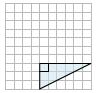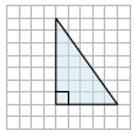# Finding the area of a right triangle on a grid

#### Complete Python Prime Pack

9 Courses     2 eBooks

#### Artificial Intelligence & Machine Learning Prime Pack

6 Courses     1 eBooks

#### Java Prime Pack

9 Courses     2 eBooks

In this lesson, we find the area of a right triangle on a grid. We draw the given right triangle on the grid and then measure its base and height on the grid. The formula for the area of triangle is used as follows to find area.

Area of triangle = $\mathbf{\frac{1}{2}}$ base × height

Find the area of the given right triangle on a grid.### Solution

Step 1:

Area of Triangle = $\frac{1}{2}$ × b × h; b = base = 6; h = height = 3

Step 2:

Area of given right triangle on a grid = $\frac{1}{2}$ × 6 × 3 = 9 square units

Find the area of the given right triangle on a grid.### Solution

Step 1:

Area of Triangle = $\frac{1}{2}$ × b × h; b = base = 5; h = height = 7

Step 2:

Area of given right triangle on a grid = $\frac{1}{2}$ × 5 × 7 = 17.5 square units